Courses

# Test: Finite Automata: Regular Languages- 2

## 15 Questions MCQ Test Question Bank for GATE Computer Science Engineering | Test: Finite Automata: Regular Languages- 2

Description
This mock test of Test: Finite Automata: Regular Languages- 2 for Computer Science Engineering (CSE) helps you for every Computer Science Engineering (CSE) entrance exam. This contains 15 Multiple Choice Questions for Computer Science Engineering (CSE) Test: Finite Automata: Regular Languages- 2 (mcq) to study with solutions a complete question bank. The solved questions answers in this Test: Finite Automata: Regular Languages- 2 quiz give you a good mix of easy questions and tough questions. Computer Science Engineering (CSE) students definitely take this Test: Finite Automata: Regular Languages- 2 exercise for a better result in the exam. You can find other Test: Finite Automata: Regular Languages- 2 extra questions, long questions & short questions for Computer Science Engineering (CSE) on EduRev as well by searching above.
QUESTION: 1

### Which of the following statements are true?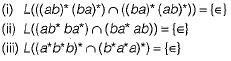Solution:

For (i) intersection of (ab)*(ba)* and (ba)*(ab)* is not {∈}. For eg. “ab” is one more string which satisfies '∩'.
Therefore (ii) is false.
For (ii) let L1 = (ab*ba*) and L2 = (ba*ab). The string in L1 should always start with ‘a’, where as for L2 it always starts with ‘b'. ∈ is not part of either language. Hence intersection is {}.
Hence (ii) is also false.
For (iii) both (a*b*b)* and (b*a*a)* contain “∈".
No other string is common. Because strings in L1 must end with ‘b ’, where a s strings in L2 must end with ‘a’.

QUESTION: 2

### Which of the following statements are true?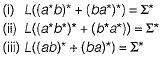Solution:

The first one is not ∑* because ‘aa’ is not present in the language L in (ii) all possible strings are present since, (a*b*)'= (a*+ b*)* = (a + b)* Therefore (ii) is ∑*. (iii) is not ∑* because, in (iii) single letter strings ‘a’ or ‘b’ are not present. Also abba, aa, bb, etc. are also not present.

QUESTION: 3

### If a DFA is represented by the following transition table, then how many states does the corresponding minimal DFA contains?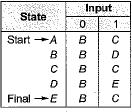Solution:

Drawing the DFA corresponding to the given transition table: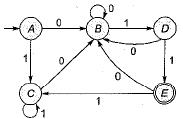Use DFA minimization algorithm
The corresponding minimum state DFA for this language can be constructed as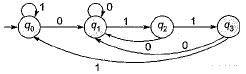Only 4 states are needed.

QUESTION: 4

Consider G = {(a, b), {S}, S, |S→b|Sa|aS|SS}) Which of the following are true?
(i) aabbaa ∈ G
(ii) G is ambiguous
(iii) Regular expression corresponding to G is ba*

Solution:

Two derivation trees are possible for aabba as given below: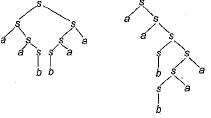Therefore (i) and (ii) are both true.
Choice (iii): ‘aba’ is also accepted by the given grammar. Therefore (iii) is false.

QUESTION: 5

Which languages does the following DFA accept?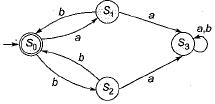Solution: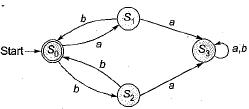We can clearly see that any number of loops of "ab” and "bb” can be accepted and ‘0’ is the accepting state.

QUESTION: 6

Which of the following statements are false?

Solution:

The given regular expression 00(1 + 10)* is not complete to denotes all strings of 0’s and 1 ’s with atleast two consecutive 0’s.
The correct regular expression must be
(0 + 1)* 00(0 + 1)*

QUESTION: 7

Which of the following statement must always be true for A and B? Suppose A and B are two sets of strings from ∑*, such that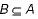(i) if A is finite then, B is finite.
(ii) If A is regular then, S is regular.
(iii) If A is context free then, B is context free,

Solution:

it is possible to have a subset of regular language which is not regular and a subset of CFL which is not CFL, but a subset of finite set has to be finite.
For example: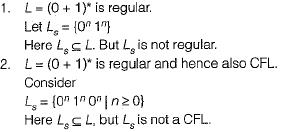Hence, (ii) and (iii) are incorrect.

QUESTION: 8

Consider the following grammar G: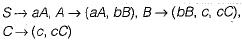Which of the following is L(G)?

Solution:

The given grammar is right linear.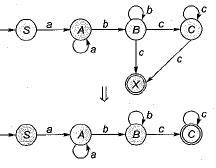The above FA accepts the language
L(G) = aa* bb* cc*

QUESTION: 9

Deterministic finite automata of a language over alphabets {0, 1), which does not contain 3 consecutive 0’s. Minimum how many states, S, in all, the DFA will have and how many of them will be final states, F?

Solution:

The regular expresession for the language:
L = {W | W does not contain 3 consecutive 0 ’s} is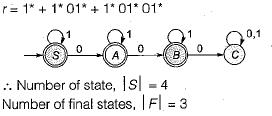QUESTION: 10

Consider the following regular grammar: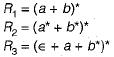Minimized deterministic finite automata of which R1, R2 and R3 are exactly same except state names?

Solution: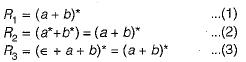Clearly from (1), (2) and (3).

QUESTION: 11

Consider the following machine M: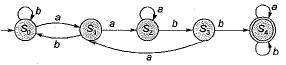What is the language L(M) accepted by this machine?

Solution: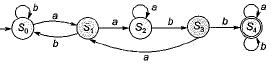Clearly, we can observe that the minimum string accepted is,aabb.
The corresponding L(M) = { w | w∈ (a + b)* aabb (a + b)*}.

QUESTION: 12

Regular grammar is

Solution:

Regular grammar is a subset of context free grammar.

QUESTION: 13

Let r1 = (a + b2)*, r2 = (a* + b*)*, r3 = (a2 + b)*. Which of the following is true?

Solution: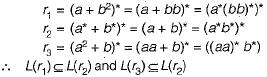QUESTION: 14

r1 = {b* ab* ab* ab*)*, r2 = (b* ab* ab*)*. What is L(r1) ∩ L(r2)?

Solution: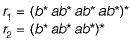r1, denotes multiple of 3 a’s, with any number of b’s.
r2 denotes multiple of 2 a’s, with any number of b’s. L(r1) ∩ L(r2) denotes multiple of 6 a’s, with any number of b’s. Hence,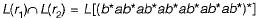QUESTION: 15

Which of the following statement is not correct?

Solution:

Since L is accepted by TM, which halts on every W in L, it is recursive. The complement must also be than recursive.